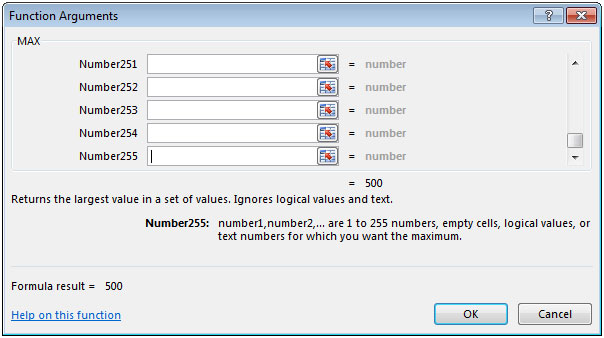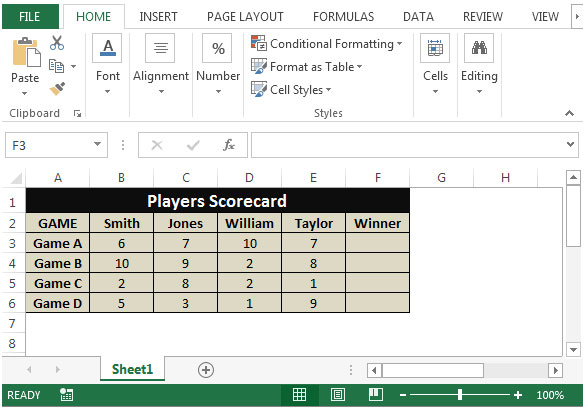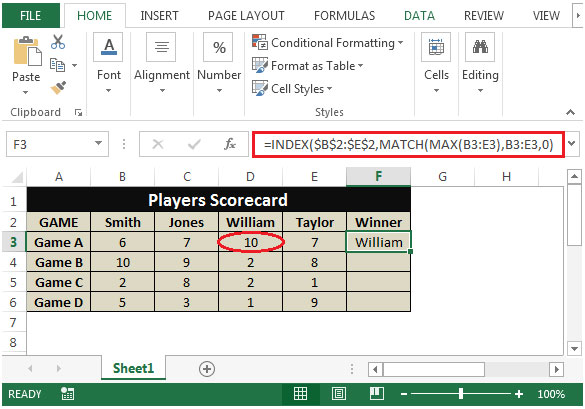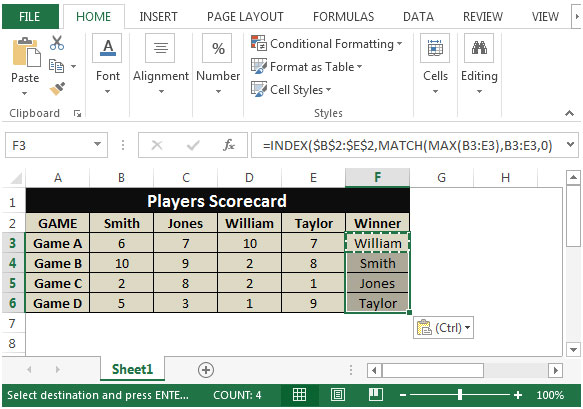# Retrieving the Column Header of the Largest Value in a Row in Excel 2010

In this article we will learn how we can retrieve the column header of the largest value in a row in Excel 2010.

If you want to recover the column header of the largest value in a row, you can use a combination of "INDEX", "MATCH" & "MAX" functions to extract the output.
"INDEX": Returns a value or reference of the cell at the intersection of a particular row and column, in a given range.

Syntax: =INDEX(array,row_num,column_num)
"MATCH" function searches for a specified item in a selected range of cells, and then returns the relative position of that item in the range.

Syntax =MATCH(lookup_value,lookup_array,match_type)
"MAX": Returns the maximum number from a range of cells or array. For example,if a list of numbers contains 5, 6, 7, 8, 9 & 10, then the output will be 10.

Syntax =MAX(number1,number2,...)

There can be a maximum 255 arguments. Refer below shown screenshot:Let us take an example:

We have 4 players scorecard for 4 games. Column A contains 4 Games while column B to E contain scores of 4 players. In column F, we need to find the winner of each row out of the scores of 4 players.• In cell F2, the formula would be
• =INDEX(\$B\$2:\$E\$2,MATCH(MAX(B3:E3),B3:E3,0))• By copying the formula from cell F2 to range F3:F6, we will get high scorer in every row.This is the way we can retrieve the column header of the largest value in a row in Microsoft Excel by using the Index, Match and Max function in combination.

1.Could you show how to find the winner if they were listed by rows instead of columns? Thanks!

2.Find result in column D from MAX in column A. Row 3 is a projected payment, alphanumeric code is placed in column A when cleared thru the bank. 9AB is the MAX, 750.00 is result.
A B C D
1 9AA DEP 1000.00 1000.00
2 9AB 1078 -250.00 750.00
3. ACH - 30.00 720.00

•Sorry, this didn’t post as I typed it. I need a formula for the previous post. MAX wont find alphanumeric. Only numbers. I tried MAXA, didn’t work on excel mobile or 360.

3.What happens if there is a tie ? Is there a way to report 2 or more scorers if they have equal scores ?

4.Can you plz show me how this is done using the
IF and MAX function

Terms and Conditions of use

The applications/code on this site are distributed as is and without warranties or liability. In no event shall the owner of the copyrights, or the authors of the applications/code be liable for any loss of profit, any problems or any damage resulting from the use or evaluation of the applications/code.